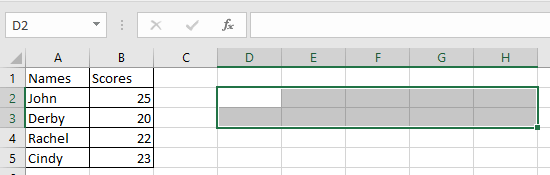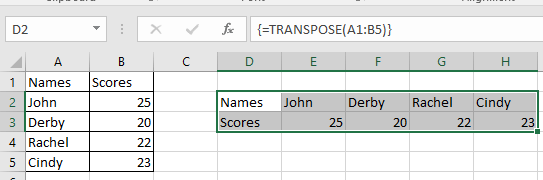# How to use the Excel TRANSPOSE function

In this article, we will learn about how to use the TRANSPOSE function in Excel.

Let’s assume there is a matrix or array of 2 x 3. It means that the matrix has 2 rows and 3 columns. But when you take the transpose of the 2 x 3 matrix. It returns the matrix of 3 x 2 by interchanging rows to columns and vice versa

The TRANSPOSE function returns the transpose of the matrix or array.
Syntax:

=TRANSPOSE(array)

Note: You need to select the cells first and then type the formula. If you select more cells than you should have. It will generate errors in the extra cells.

Let’s understand this function using it an example.
Here we have numbers in an array. we need to find the transpose of the arrayFirst of all we need to select the exact columns and rows the same as the rows and columns
Use the formula in the selected cells

{=transpose(A1:B5)}

Use Ctrl + Shift + Enter instead of just Enter to get the curly braces. DO NOT put curly braces manuallyAs you can see the rows become columns and columns becomes rows. This function gets you the transpose of the matrix or array..

Hope you understood how to use TRANSPOSE function in Excel. Explore more articles on Excel mathematical function function here. Please feel free to state your query or feedback for the above article.

Related Articles:

How To Switch Rows and Columns in Excel

Unhide multiple Rows

Popular Articles:

How to use the VLOOKUP Function in Excel

How to use the COUNTIF function in Excel 2016

How to Use SUMIF Function in Excel

Terms and Conditions of use

The applications/code on this site are distributed as is and without warranties or liability. In no event shall the owner of the copyrights, or the authors of the applications/code be liable for any loss of profit, any problems or any damage resulting from the use or evaluation of the applications/code.